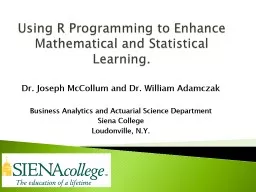# Using R Programming to Enhance Mathematical and Statistical Learning.Embed code:

## Using R Programming to Enhance Mathematical and Statistical Learning.

Download Presentation - The PPT/PDF document "Using R Programming to Enhance Mathemati..." is the property of its rightful owner. Permission is granted to download and print the materials on this web site for personal, non-commercial use only, and to display it on your personal computer provided you do not modify the materials and that you retain all copyright notices contained in the materials. By downloading content from our website, you accept the terms of this agreement.

### Presentations text content in Using R Programming to Enhance Mathematical and Statistical Learning.

Slide1

Using R Programming to Enhance Mathematical and Statistical Learning.

Dr. Joseph McCollum and Dr. William AdamczakBusiness Analytics and Actuarial Science DepartmentSiena CollegeLoudonville, N.Y.

Slide2

Poor ability with Order of Operations.Difficulty with notation.Poor ability of students when it comes to logic and algorithmic thinking.

Taking the time to think through a problem.Acceptance of the “black box” answer.Weaknesses of Students

Slide3

How coding in

R helps: A function to calculate standard deviation or correlation cannot be built without first understanding the order of events which leads to an understanding of the notation. This understanding is not available when using prepacked functions.Writing Code to Overcome the Weaknesses

Slide4

Averagefunction <- function(x){Sum(x)/length(x)

}Zvaluefunction <- function(x, dec=TRUE){Ifelse(dec,abs(qnorm((1-x)/2)), “Error”)}

Warm Up Exercises

Slide5

Example 1:

stdev <- function(x, sample=TRUE) { denom <- ifelse(sample,length(x)-1,length(x)) mean <- sum(x)/length(x) numer <- sum((x-mean)^2) sqrt(numer/denom)

}

Ensuring

S

tudents

U

nderstand Standard Deviation

Slide6

Students do covariance.Students do correlation.Students build a Confidence Interval for Mean with Population Standard Deviation Known. This is a great example to talk to students about default values.

Extension to Learning

Slide7

bobCI <- function(x, ci=0.95, stp=1){ mean <-sum(x)/length(x) zvalue

<-abs(qnorm((1-ci)/2)) serror <-stp/sqrt(length(x)) lb <-mean - zvalue * serror ub <-mean + zvalue * serror

ans

<-c(

lb,ub

)

return(

ans

)

}

CI for Mean and Sigma Known

Slide8

Using Code/Functions as a Framework

Slide9

Example 2: t.test in Rt.test

(x, y = NULL, alternative = c("two.sided", "less", "greater"), mu = 0, paired = FALSE, var.equal = FALSE, conf.level = 0.95, …)The arguments taken by t.test provide a framework for selecting the correct test based on the context of the problem and address all considerations. Using Code/Functions as a Framework

Slide10

Load the dataset called Golf and you will have a sample of 33 golfers who competed in the 2017 Masters. You will have each golfer’s first round and final round score.Question 1: Use a 1% level of significance to determine if the population mean score for the first round is greater than 70?

Answer 1: t.test(Round1, alternative=“greater”,conf.level=0.99)Question 2: Use a 5% level of significance to determine if there is a statistically significant difference between the population means of the first and final round score?Answer 2: t.test(Round1, FinalRound,paired=TRUE)

Example done with

t.test

Slide11

Scenario: A student is doing their homework on the nonparametric method called Mann-Whitney-Wilcoxon Rank Sum Test for large samples. They are doing well in class and so just quickly enter in R the code (wilcox.test) and they do not get the GREEN checkmark on the online homework program.

We all know what happens next. Late night emails to the professor.I Just Want the Answer!

Slide12

R programming is great but since it is open source we have to every now and then check to see if the answers make sense and why.In our scenario the textbook we use from Pearson by Berenson utilizes an alternative version of the Wilcoxon test.

As I teach the differences and similarities between the two versions I have a chance to address the student weaknesses again.Be Careful of the “Black Box”

Slide13

1) Introducing and writing basic function codes in R has helped our students with their weaknesses in our statistic classes.2) Utilizing pre-programmed functions like (

t.test) has helped our students slow down and think logically through a problem.3) We make sure the students understand the answer and not just trust the “black box”.Review

Slide14

Thank You!!!

Slide15

EXTRA SLIDES

Slide16

-A private Catholic college in upstate N.Y.

-Population is around 3200 students-Average class size is around 25 students

-Percent of the students in the Business School is around 40%

-BAAS department teaches about 1400 students a year

Siena College

Slide17

BAAS department is a part of the School of Business

BAAS teaches 2 pre-business required foundational math courses of Financial Math and Business CalculusBAAS teaches 2 business core courses of Business Statistics and Operations ManagementAll students in the School of Business are required to take two Computer Science courses but they do not have to be taken before Statistics.

Business Analytics and Actuarial Science Department (BAAS)

Slide18

Σ( ) = sum( )n = length( )Ifelse

(condition, result if T, result if F)Introduction into R and Notation

Slide19

T1 = sum of the ranks assigned to the n1 values in the smaller sample.T2 = sum of the ranks assigned to the n2 values in the larger sample.Fact: T1+T2 = (.5)(n)(n+1)Book uses T1.

R uses W = T1 – (.5)(n1)(n1+1).Both arrive at the same conclusion when dealing with a large sample.Wilcoxon Rank Sum Test

Slide20

Z = (T1 – [.5*(n1)(n+1)])/SEWhere SE = Sqrt[(1/12)*(n1)(n2)(n+1)]And

Z=(W – (.5)(n1)(n2))/SEWith same SEWicoxon Rank Sum Test Part II